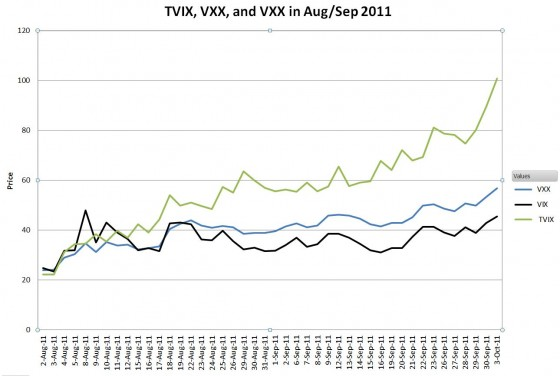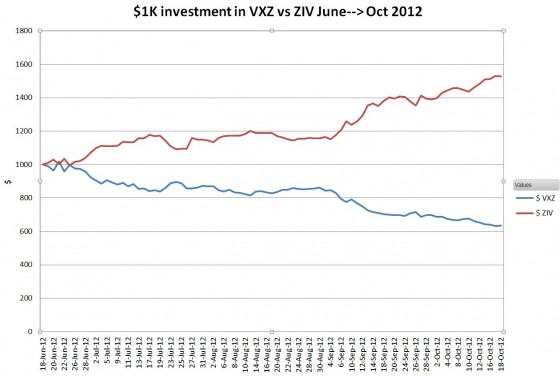# Sometimes Inverse / Leveraged Volatility Funds Outperform Their Leverage Factors

From August 2nd  to October 3rd, 2011 Barclays’ S&P 500 VIX Short Term Futures ETN (VXX) had a great 137% runup.  In that same period VelocityShares’ TVIX  ETN, 2X leveraged on the same index went up an astonishing 348%,  73 percent more than its 2X leverage factor would project.   How is that possible?  Don’t inverse and leveraged funds always underperform the index they’re tracking?

Normal market price action is typically random, with the number of up and down days about equal.   This bouncing back and forth is really bad for inverse and leveraged funds.  A classic example is a two day sequence of 10% up one day followed by a 9.091% move down.

 Fund Day 1 (+10%) Day 2 (-9.091%) Gain / Loss (excess) 1X (underlying) 100 * (1+ 0.1) = 110 110 * (1-0.9091) = 100 0% -1X (inverse) 100* (1-0.1)      = 90 90*(1+.09091) = 98.18 -1.8 % (1.8% loss) 2X 100 * 1+2*(0.1)) =120 120*(1+2*(-0.09091) = 98.18 -1.8 % (1.8% loss) 3X 100 * 1+3*(0.1)) =130 130*(1+3*(-.09091) = 94. 55 -5.45% (5.45% loss)

The non-leveraged fund ends up unchanged.  But all the daily rebalanced -1X, 2X and 3X leveraged funds suffer.  For more on rebalancing see Under the hood of a leveraged ETF.

There wasn’t much back and forth for TVIX in the Aug/Sept 2011 timeframe.The average daily increase in VXX was 2% —which went on for 44 trading days.   Looking at just 3 days of ongoing 2% gains we get the following results:

 Fund Day 1 (+2%) Day 2 (+2%) Day 3 (+2%) Gain / Loss (excess) 1X (underlying) 100 * (1+ 0.02) = 102 102 * (1+ 0.02) = 104 104 * (1+ 0.02) = 106.1 6.1% overall gain -1X (inverse) 100* (1-0.02)      = 98 98* (1-0.02)      = 96.04 96.04* (1-0.02)     = 94.12 5.88% loss         (0.24% excess) 2X 100 * 1+2*(0.02)) =104 104 * 1+2*(0.02)) =108.16 108.16 * 1+2*(0.02)) =112.49 12.49% overall gain (0.24% excess) 3X 100 * 1+3*(0.02)) =106 130 * 1+3*(0.02)) =112.36 112.36 * 1+3*(0.02)) =119.1 19.1% overall gain (0.8% excess)

When carried out for 44 days the projected excess gain for TVIX given a constant +2% daily change in VXX gives a theoretical excess of 288%.  The actual excess gain was “only” 73% because VXX did have a few down days along the way.

During this timeframe leveraged funds had 3 things going for them:

1. Volatility as measured by VIX trending higher
2. Backwardation in the VIX futures was boosting VXX
3. The increases in VXX were steady, without a lot of random motion—resulting in a positive compounding effect.

These three conditions are relatively rare, happening roughly once every two years.

On the other hand, these three conditions are considerably more common:

1. Volatility as measured by VIX is trending down
2. The VIX futures term structure is in contango
3. The decrease in volatility is steady, without a lot of randomness—resulting in a positive compounding effect.

These conditions were satisfied from mid-June to mid-October in 2012, and the performance of inverse volatility funds was impressive.  The chart below shows \$1K invested in Barclays’ medium term volatility product VXZ, and ZIV, VelocityShares’ -1X daily reset medium term volatility ETN.VXZ went down 36.4% during this 3 month period, ZIV was up 52.7%.  This hat trick on inverse volatility paid off nicely.

First posted on

### 6 thoughts on “Sometimes Inverse / Leveraged Volatility Funds Outperform Their Leverage Factors”

You have a great site, l look forward to following.

• Thanks Ted!

— Vance

2. Leveraged and inverse funds should always underperform the corresponding multiple of the underlying index in log returns. So if VXX went up 137%, i.e. 2.37x, we would have expected TVIX to have gone up 2.37^2 or 5.62x or 462% if it had no underperformance. Since TVIX actually went up only 348%, that’s an underperformance of 114%.

• I don’t know where your square term on the multiplier comes from (2.37^2). Daily rebalanced 2X leverage funds only target twice the daily percentage change on the underlying. . The reasonable / simplistic extension would be twice the percentage gain over a longer time frame, not twice (or square of) the multiplier. Of course, even this bar is rarely met due to path dependencies/compounding. This post points out an exception to that norm.